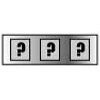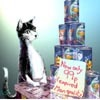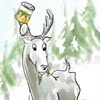#### You may also like### Forgotten Number

I have forgotten the number of the combination of the lock on my briefcase. I did have a method for remembering it...### Human Food

Sam displays cans in 3 triangular stacks. With the same number he could make one large triangular stack or stack them all in a square based pyramid. How many cans are there how were they arranged?### Sam Again

Here is a collection of puzzles about Sam's shop sent in by club members. Perhaps you can make up more puzzles, find formulas or find general methods.

# Triangle Numbers

##### Age 11 to 14 Challenge Level:

The first triangle number is $1$
The second triangle number is $1 + 2$
The third triangle number is $1 + 2 + 3$
The fourth triangle number is $1 + 2 + 3 + 4 \ldots$

The $n^{\text{th}}$ triangle number is $\frac{n(n+1)}{2}$